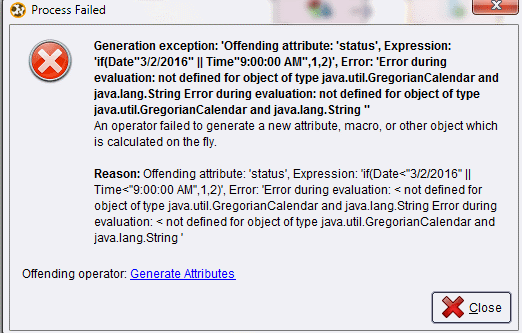Convert and compare - Date and Time

Member Posts: 30Contributor I
edited November 2018 in Help

Hi,

I have a dataset with 2 columns (Date and Time):

Date showing "1/1/2016", "23/4/2016".

Time showing "12:04:06 PM", "7:12:23 AM".

Question: I would like to show that any data with date (earlier than 3/2/2016) or time (earlier than 9:00:00 AM), a status will show "1" else show "2", I tried below but having error. May I know which area I have done wrongly and can the display be kept as original?

====

1. I import the data and noticed that the data type is polynominal for the date and time.

2. Thus,  I used the data transformation "Nominal to Date":

a) For date, i convert to "date" data type with date format as "dd/mm/yyyy"

b) For time, I convert to "time" data type with date format as "hh:mm:ss a"

3. I used generate attribute to do comparison.

a) attribute name: status

b) function expression: if(Date<"3/2/2016" || Time<"9:00:00 AM",1,2)

However, I received the following error:Tagged:

• RapidMiner Certified Analyst, RapidMiner Certified Expert, Member Posts: 1,761Unicorn

I think you need to use the eval() function for this, which I don't think is available in the version you are using. It appears you are using v5.3?

• Member Posts: 1Contributor I
Hi,

I have three Attribute Day, Month, and Year. i want to get it like 22/12/2018. do we have any function for it?

• Administrator, Moderator, Employee, RapidMiner Certified Analyst, RapidMiner Certified Expert, Community Manager, RMResearcher, Member, University Professor Posts: 1,597RM Founder
Hi,
Sure, you can use a combination of the operators Generate Attributes and Nominal to Date.  Below is an example process showing this.
Hope this helps,
Ingo
``<?xml version="1.0" encoding="UTF-8"?><process version="9.2.001">  <context>    <input/>    <output/>    <macros/>  </context>  <operator activated="true" class="process" compatibility="9.2.001" expanded="true" name="Process">    <parameter key="logverbosity" value="init"/>    <parameter key="random_seed" value="2001"/>    <parameter key="send_mail" value="never"/>    <parameter key="notification_email" value=""/>    <parameter key="process_duration_for_mail" value="30"/>    <parameter key="encoding" value="UTF-8"/>    <process expanded="true">      <operator activated="true" class="utility:create_exampleset" compatibility="9.2.001" expanded="true" height="68" name="Create ExampleSet" width="90" x="45" y="34">        <parameter key="generator_type" value="comma separated text"/>        <parameter key="number_of_examples" value="100"/>        <parameter key="use_stepsize" value="false"/>        <list key="function_descriptions"/>        <parameter key="add_id_attribute" value="false"/>        <list key="numeric_series_configuration"/>        <list key="date_series_configuration"/>        <list key="date_series_configuration (interval)"/>        <parameter key="date_format" value="yyyy-MM-dd HH:mm:ss"/>        <parameter key="time_zone" value="SYSTEM"/>        <parameter key="input_csv_text" value="Day, Month, Year&#10;19, 08, 1977"/>        <parameter key="column_separator" value=","/>        <parameter key="parse_all_as_nominal" value="false"/>        <parameter key="decimal_point_character" value="."/>        <parameter key="trim_attribute_names" value="true"/>      </operator>      <operator activated="true" class="generate_attributes" compatibility="9.2.001" expanded="true" height="82" name="Generate Attributes" width="90" x="179" y="34">        <list key="function_descriptions">          <parameter key="Date" value="Day+&quot;/&quot;+Month+&quot;/&quot;+Year"/>        </list>        <parameter key="keep_all" value="true"/>      </operator>      <operator activated="true" class="nominal_to_date" compatibility="9.2.001" expanded="true" height="82" name="Nominal to Date" width="90" x="313" y="34">        <parameter key="attribute_name" value="Date"/>        <parameter key="date_type" value="date"/>        <parameter key="date_format" value="dd/MM/yyyy"/>        <parameter key="time_zone" value="SYSTEM"/>        <parameter key="locale" value="English (United States)"/>        <parameter key="keep_old_attribute" value="false"/>      </operator>      <connect from_op="Create ExampleSet" from_port="output" to_op="Generate Attributes" to_port="example set input"/>      <connect from_op="Generate Attributes" from_port="example set output" to_op="Nominal to Date" to_port="example set input"/>      <connect from_op="Nominal to Date" from_port="example set output" to_port="result 1"/>      <portSpacing port="source_input 1" spacing="0"/>      <portSpacing port="sink_result 1" spacing="0"/>      <portSpacing port="sink_result 2" spacing="0"/>    </process>  </operator></process>``
• Member Posts: 497Unicorn
edited April 13
Hello @sshah8050

You can concatenate these three attributes (attributes should be of type nominal) using Generate attributes operator into a single attribute and then parse it (if you need it as a date datatype)  into a date using Nominal to date operator. Sample XML code below.

<?xml version="1.0" encoding="UTF-8"?><process version="9.2.001">
<context>
<input/>
<output/>
<macros/>
</context>
<operator activated="true" class="process" compatibility="9.2.001" expanded="true" name="Process">
<parameter key="logverbosity" value="init"/>
<parameter key="random_seed" value="2001"/>
<parameter key="send_mail" value="never"/>
<parameter key="process_duration_for_mail" value="30"/>
<parameter key="encoding" value="SYSTEM"/>
<process expanded="true">
<operator activated="true" class="retrieve" compatibility="9.2.001" expanded="true" height="68" name="Retrieve DataSet" width="90" x="112" y="85">
<parameter key="repository_entry" value="//Local Repository/RapidMIner/DataSet"/>
</operator>
<operator activated="true" class="generate_attributes" compatibility="9.2.001" expanded="true" height="82" name="Generate Attributes" width="90" x="447" y="85">
<list key="function_descriptions">
<parameter key="Date" value="concat(SURVEY,&quot;/&quot;,DOEID,&quot;/&quot;,WALLTYPE)"/>
</list>
<parameter key="keep_all" value="true"/>
</operator>
<operator activated="true" class="nominal_to_date" compatibility="9.2.001" expanded="true" height="82" name="Nominal to Date" width="90" x="648" y="85">
<parameter key="attribute_name" value="Date"/>
<parameter key="date_type" value="date"/>
<parameter key="date_format" value="yyyy/MM/dd"/>
<parameter key="time_zone" value="SYSTEM"/>
<parameter key="locale" value="English (United States)"/>
<parameter key="keep_old_attribute" value="false"/>
</operator>
<connect from_op="Retrieve DataSet" from_port="output" to_op="Generate Attributes" to_port="example set input"/>
<connect from_op="Generate Attributes" from_port="example set output" to_op="Nominal to Date" to_port="example set input"/>
<connect from_op="Nominal to Date" from_port="example set output" to_port="result 1"/>
<portSpacing port="source_input 1" spacing="0"/>
<portSpacing port="sink_result 1" spacing="0"/>
<portSpacing port="sink_result 2" spacing="0"/>
</process>
</operator>
</process>

Thanks
Regards,
Varun